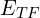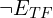# Advanced (yet simple) Prompt Engineering techniques for Large Language Models

Prompt Engineering, in the context of Large Language Models (LLMs), refers to the techniques that optimize the input prompt to enhance the performance of the models’ output. In this article, we introduce simple prompt engineering methods that are proven to work well in various domains.

## Few-Shot Examples

Domains of application: Few-shot examples are helpful in almost every field. We should put a few examples in the input for LLMs whenever possible.

Idea: The examples help the LLMs understand the problem to solve and illustrate how the output should look like.

Example: An illustration of few-shot examples is shown in the figure below, where the task is to translate texts from English to French. Specifically, we want to translate “cheese” into French. To do that, we prepare a prompt as shown in the figure. In the beginning, the task description is given, then, there are a few (three) examples of translation, and finally, the word we want to be translated (“cheese”) followed by “=>” as a separator between English and French. Intuitively, the LLMs will generate “fromage” as the output.

## Chain of Thought (CoT)

Domains of application: Chain-of-Thought prompting has been shown to boost LLMs’ performance in arithmetic, commonsense, and symbolic reasoning tasks . The larger the LLMs, the more benefits CoT brings in. For models with fewer than 100B parameters, CoT does not yield any performance gain.

Idea: Tasks that require logical inference may be hard for LLMs to give correct answers right away. CoT prompting allows the LLMs to simulate the reasoning process of humans before giving an answer at the end.

Example: The figure below exemplifies how to use CoT. While in the standard prompt, the answer in the example only splits out the final results; the answer in the CoT prompt also includes step-by-step reasoning of how to come up with the result (the part in blue). This instructs the LLMs to reason before giving the result for the testing example (the part in green).

## Self-Consistency

Domains of application: The authors have done experiments with Self-Consistency and gotten good results on arithmetic, commonsense, and symbolic reasoning tasks . Intuitively, this technique is probably helpful for all the tasks whose final results can be aggregated automatically.

Idea: Instead of asking the LLMs to generate one answer, it is better to ask for multiple answers and take the one that is most common among them. Note that an LLM can give different answers to the same input if its parameter controlling the creativity (or technically, the temperature) is set to a positive value (rather than 0).

Example: The Self-Consistency technique is illustrated in the figure below. Notice that few-shot examples and Chain-of-Thought are also used in the prompts. On the top of the figure is the CoT prompt, which gives an incorrect answer. On the bottom, Self-Consistency is applied by sampling multiple answers from the LLM using the same prompt as the one on top; since the majority of the answers are “\$18”, this answer is selected as the final answer (and yes, it is correct).

## Ask Me Anything (AMA) prompt

Domains of application: Ask Me Anything prompting was shown to boost LLMs’ performance on diverse language tasks, including SuperGLUE, NLI, classification, and QA — 20 tasks in total .

Idea: AMA improves upon existing prompting techniques by introducing 2 essential changes. One is to replace restrictive questions (e.g., “John went to the park. True or False?”) with open-ended questions (e.g., “Who went to the park?”) – this makes sense because open-ended questions and answers are more prevalent in the training data of the LLMs as compared to restrictive ones. The other change has some similarities with Self-Consistency: they ask for multiple answers from the LLM before aggregating them to get the final prediction. However, in contrast to Self-Consistency which uses the majority voting scheme, AMA combines the answers using Weak Supervision. That is, they compute a weight for each answer, where the weights are based on the covariance between the prompts generating those answers – two prompts having very high covariance will make the weights of the two corresponding answers low, and select the answer with the highest weighted sum. More details can be found in the paper.

Example: The figure below illustrates the AMA prompting with an example. In the first step, the restrictive True/False question is converted to multiple different open-ended questions. These (open-ended) questions are generated by the LLM using different few-shot examples. Each of these questions is then asked to get an answer from the LLM independently. In the second step, the answers are aggregated by Weak Supervision, as described above, to output the final prediction.

## Maieutic prompt

Domains of application: Commonsense reasoning and fact verification tasks . Ideally, the output of the task should be a True/False answer.

Idea: Maieutic prompting attempts to validate an (LLM-generated) answer by making sure the LLM is consistent on this answer and the rationale (or explanation) behind it. For example, given a statement S, the LLM says S is True because of some explanation E; However, if given E, the LLM says E is False, then we should not believe its first conclusion that S is True.

Example: Below is an illustration of Maieutic prompting for an exemplar reasoning question. Given the proposition “War cannot have a tie”, they build a Maieutic tree from the answers of the LLM. Here, a proposition, for example,, is logically Integral (the orange part) if the LLM consistently infers the truth value ofand. The tree is extended until all leaves are logically integral. Naturally, we have relations (Entail or Contradict) between the pairs of propositions. Furthermore, they assign weights to propositions and the relations, before using a symbolic Max-SAT Solver to deduce the final prediction for the original proposition (War cannot have a tie? False!).

## AI Chains

Domains of application: Tasks that can be broken down into smaller tasks.

Idea: Given a complex task, the idea of AI Chains is to break it down into subtasks, where the subsequent subtask(s) may be dependent on the result of the prior subtask(s) .In particular, they manually pre-define 8 primitive operations such that each can be adequately handled by a single LLM run. Instead of asking the LLM to give an answer for the complex task all at once, they use multiple queries, each for one of the easier subtasks (or operations).

Example: The example in the figure below showcases how AI Chaining is used (B) as compared to no chaining (A) for the task of rewriting feedback for a presentation. Particularly, they split the original task (rewriting feedback) into 3 subtasks: 1. extracting individual presentation problems from the original feedback; 2. discussing suggestions for each problem; and 3. composing new, better feedback based on the problems and suggestions.

## Least-to-Most prompt

Domains of application: This technique is shown to perform well on symbolic manipulation, compositional generalization, and math reasoning tasks . In these experiments, Least-to-most prompting improved the LLMs’ performance when the few-shot examples in the prompt are easier (or less complex) than the testing instance.

Idea: Given a complex problem, we apply a 2-stage procedure: 1. problem break-down: ask the LLM to reduce the problem into multiple sub-problems; 2. sequential solving: ask the LLM to solve the sub-problems sequentially, where the answer for the next subproblem is built on the answer of the previous ones.

Example: The figure below illustrates the 2 stages of Least-to-most prompting on a math word problem. In stage 1, the LLM creates a simpler subproblem. In stage 2, the LLM sequentially gives answers for the subproblem and the original problem augmented by the subproblem together with its answer.